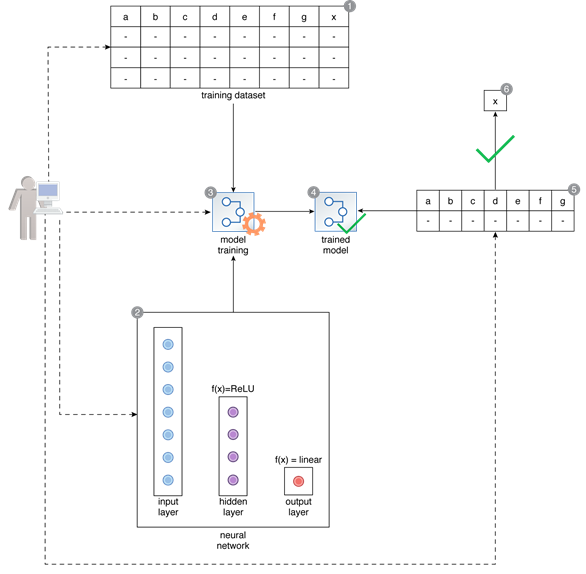# Supervised Network Configuration

Artificial Intelligence (AI) Patterns, Neurons and Neural Networks > Supervised Learning Patterns > Supervised Network Configuration

## Supervised Network Configuration

How can neural networks be constructed to solve regression and classification problems?

### Problem

All neural networks are generally built around some variation of input, hidden and output layers including linear and non-linear transformations. However, without knowing how to configure the different layers or which non-linear functions to use, constructing a network can take a long time and can produce invalid or incomprehensible results.

### Solution

For regression tasks the number of neurons for the input layer is set to the number of inputs, while the output layer contains a single neuron (with Linear activation). For classification tasks, the number of neurons in the input layer is the same as the target classes (with Softmax activation). Hidden layers generally use the ReLU activation function.

### Application

The number of neurons and the activation function are configured via the neural network development library (such as TensorFlow or Keras), while specifying the architecture of the network.A data scientist prepares a training dataset that is comprised of 7 input numerical features and a numerical target feature of x (1). They need to train a 3-layer neural network to predict the value of the target feature, based on which they specify the architecture of the network by selecting 7 neurons for the input layer, 4 for the hidden layer, and 1 for the output layer, and selecting ReLU and Linear activation functions for the hidden and output layers respectively (2). After training, the trained network is fed an unseen input to predict the value of target x (3, 4, 5). By specifying the correct architecture of the network, the data scientist is able to successfully proceed with developing a solution and predicting the value of x (6).